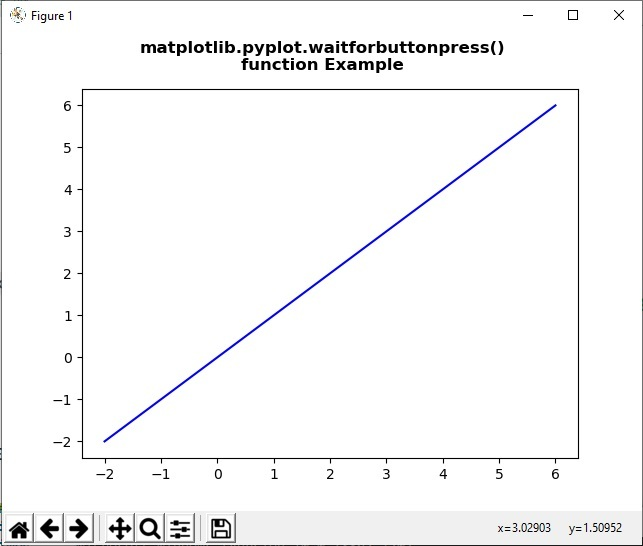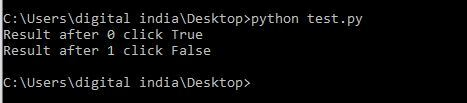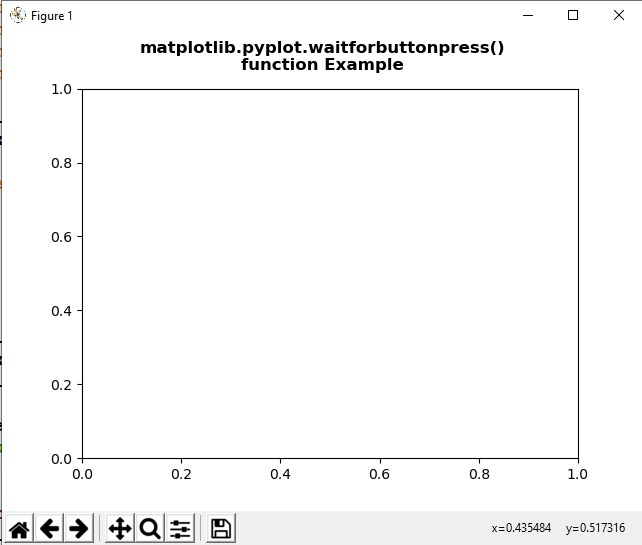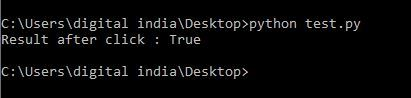Related Articles

# Matplotlib.pyplot.waitforbuttonpress() in Python

• Last Updated : 10 May, 2020

Matplotlib is a library in Python and it is numerical – mathematical extension for NumPy library. Pyplot is a state-based interface to a Matplotlib module which provides a MATLAB-like interface. There are various plots which can be used in Pyplot are Line Plot, Contour, Histogram, Scatter, 3D Plot, etc.

## matplotlib.pyplot.waitforbuttonpress() method

The waitforbuttonpress() method in pyplot module of matplotlib library is used for blocking call to interact with the figure.

Syntax: matplotlib.pyplot.waitforbuttonpress(timeout=- 1)

Parameters: This method accept the following parameters that are discussed below:

• timeout: This parameter is the timeout value.

Returns: This method does not returns any value.

Below examples illustrate the matplotlib.pyplot.waitforbuttonpress() function in matplotlib.pyplot:

Example 1:

 `# Implementation of matplotlib function ``import` `numpy as np ``import` `matplotlib.pyplot as plt `` ` ` ` `for` `ite ``in` `range``(``2``): ``    ``x ``=` `np.linspace(``-``2``, ``6``, ``100``) ``    ``y ``=` `(ite ``+` `1``)``*``x `` ` `    ``fig ``=` `plt.figure() ``     ` `    ``ax ``=` `fig.subplots() ``    ``ax.plot(x, y, ``'-b'``) `` ` `    ``fig.suptitle(``"""matplotlib.pyplot.waitforbuttonpress()``function Example\n\n"""``, fontweight ``=``"bold") `` ` `    ``w ``=` `plt.waitforbuttonpress() ``    ``print``(``"Result after"``, ite, ``"click"``, w) `` ` `    ``plt.show() `

Output:Example 2:

 `# Implementation of matplotlib function ``import` `numpy as np ``import` `matplotlib.cm as cm ``import` `matplotlib.mlab as mlab ``import` `matplotlib.pyplot as plt ``   ` ` ` `fig ``=` `plt.figure() ``ax ``=` `fig.subplots() ``   ` `def` `tellme(s): ``       ` `    ``fig.suptitle(s, fontweight ``=``"bold"``) ``    ``fig.canvas.draw() ``    ``renderer ``=` `fig.canvas.renderer ``    ``fig.draw(renderer) ``   ` `plt.clf() ``ax.axis([``-``1.``, ``1.``, ``-``1.``, ``1.``]) `` ` `plt.setp(plt.gca(), autoscale_on ``=` `False``) ``   ` `tellme(``"""matplotlib.pyplot.waitforbuttonpress()``function Example\n\n"""``) ``   ` `w ``=` `plt.waitforbuttonpress() ``print``(``"Result after click :"``, w) `` ` `plt.show() `

Output:Attention geek! Strengthen your foundations with the Python Programming Foundation Course and learn the basics.

To begin with, your interview preparations Enhance your Data Structures concepts with the Python DS Course. And to begin with your Machine Learning Journey, join the Machine Learning – Basic Level Course

My Personal Notes arrow_drop_up